# The animated company logo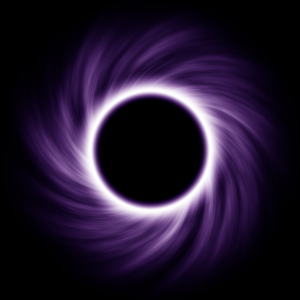You can probably see that the logo at the top left is animated. At least if your browser decided to cooperate. This was done by using a .webm (or .mp4) video instead of just a regular image.

But how was the logo made? The answer is not "with an image/video editing software a normal person would use"

## The initial concept

When deciding on a company name, one of the main consideration was if it could have a cool logo. And VOID sounds pretty cool, so I checked google images: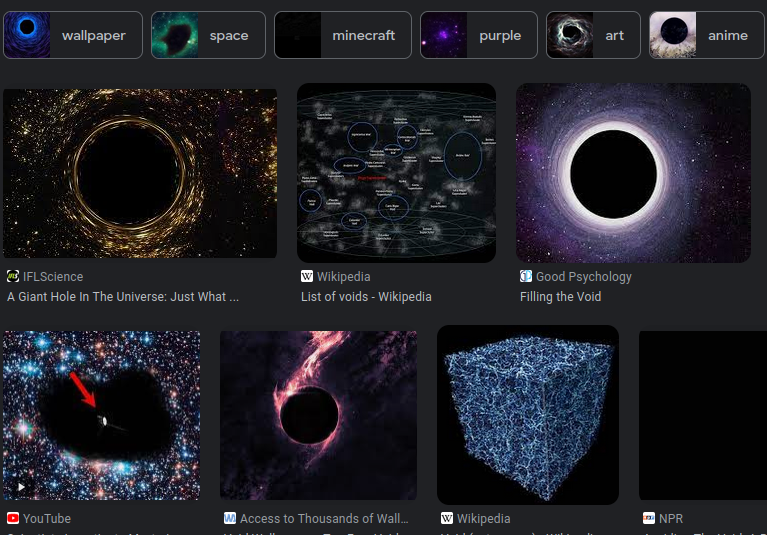Ok, basically pre-Interstellar black hole. Sounds doable, and would look good in place of the O.

## The concept art

Being the efficient person I am, I asked my wonderful wife to help me (i.e. create the logo for me). She's been playing around with Stable Diffusion lately, so I guessed it would be a few minutes of prompt engineering.

We went through some iterations, with me being the worst kind of customer ("hmm, this isn't what I wanted, can you make it bluer?" x 100), and this was the final result before she gave up: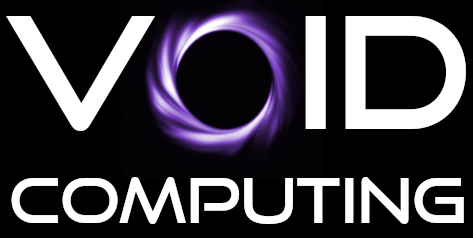As you can see I kept the font, it was a great find (and funnily enough, it was made in 1998, exactly the date where I get my retro-futuristic style ideas from).

But the swirl could be made better.

Note that this shows that the AI generators are good concept art and sketching tools, but fine tuning is basically impossible, you will always have to do manual work.

## GIMP

The next day, I decided to finally install GIMP, the vim of image editors. I hate that software, last time I used it was some 8 years ago, and gave up when I couldn't find how to make a straight line, and installed KolourPaint instead.

Turns out, GIMP is not that bad nowadays, and is actually somewhat discoverable.

I had a concept of what to do too: create a simple starburst pattern and twist it.

1. Generate some Perlin noise *
3. Convert to polar coordinates
4. Apply twist filter
5. Do some color mapping

*(I used to develop games, and once you find out about Perlin noise, you will do everything with it. It's the absolute hottest code path in Stickman Warfare )

So first step, noise. My GIMP's Perlin noise looks buggy, it leaves a kind of square pattern: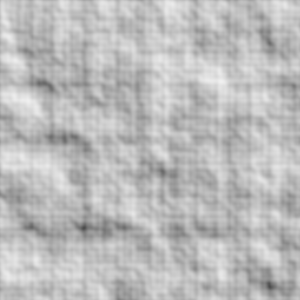I found another one, called "Solid noise" which looks very similar to what I know as Perlin noise: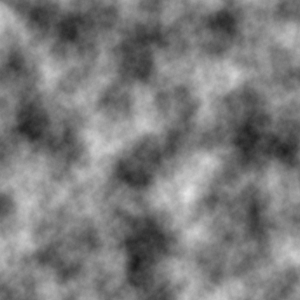Now if we stretch it out a bit and apply polar coordinates, you will see where we are going: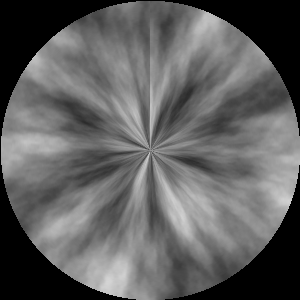Well, maybe not. Let's apply a nice custom gradient (found as an alternative tool under the paint bucket... did I ever tell you I hate GIMP?) and then redo the polar coordinates: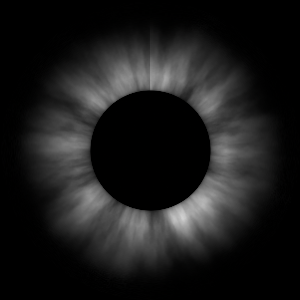Now just a "Whirl and pinch" and color mapping, and we recreated the logo concept: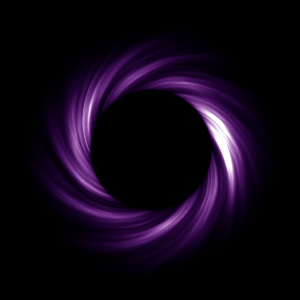Looks... OK.

Now here's a problem: if I want to tweak any of these transformations, I have to go through all of them manually. And I want to do a lot of tweaks, this is an important logo.

There are a few solutions: scripting GIMP or maybe redoing it in Blender or a similar node-based texture generator. Both sounds like a lot of learning, so

## Let's generate it in python

Or as Aphyr put it, let's pull a logo out of the void.

OK, so let's start with some scaffolding. Once you have a pypy virtualenv (otherwise the rendering takes days) and pypng installed into it, we can have a basic framework:

 ``` 1 2 3 4 5 6 7 8 9 10 11 12 13 14 15 16 17 18 19 20``` ``````import png SIZE = 300 def pixel_value(x, y): return [x, y, 0, 1] def generate_image(): return [ b"".join( bytes( round(255 * component) for component in pixel_value(x/SIZE, y/SIZE) ) for x in range(SIZE) ) for y in range(SIZE) ] png.from_array(generate_image(), 'RGBA').save('out.png') ``````

Which generates this exquisite piece of art: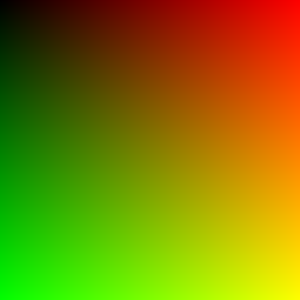`pixel_value(x,y)` is what we are going to extend here. It gets the X and Y coordinates as 0..1 ranges, and has to output R, G, B and A components as a 0..1 float. This is very similar to what pixel shaders do, and this is because I wanted a familiar environment. (This is why the above picture might look familiar. It's one of those typical shader tutorial screenshots)

Ok, so what was the next GIMP step? Ah yes, Perlin noise. I won't bore you with the details, there are a metric fsckton of Perlin noise tutorials and libraries out there. I haven't found any in python to my liking, so what I've done is rewritten the old Delphi version (which I think I've got from a gamedev forum in 2007?) in python:

 ``` 1 2 3 4 5 6 7 8 9 10 11 12 13 14 15 16 17 18 19 20 21 22 23 24 25 26 27 28 29 30 31 32 33 34 35 36 37 38 39 40 41 42 43 44 45 46 47 48 49 50 51 52 53 54 55 56 57 58 59 60 61 62 63 64 65 66``` ``````# perlin.py import math import random from typing import List TABLE_SIZE = 1024 TABLE_MASK = 1023 def complex_noise(x: float, y: float, *, x_freq: int, y_freq: int, octaves: int = 5, coarseness: float = 0.5) -> float: result = 0 multiplier = 1 for _ in range(octaves): result += noise(x * x_freq, y * y_freq, x_freq, y_freq) * multiplier x_freq *= 2 y_freq *= 2 multiplier *= coarseness return result def noise(x: float, y: float, tile_x: int, tile_y: int) -> float: ix0 = int(math.floor(x)) % tile_x ix1 = (ix0 + 1) % tile_x fx0 = x % 1 fx1 = fx0 - 1 wx = smooth(fx0) iy0 = int(math.floor(y)) % tile_y iy1 = (iy0 + 1) % tile_y fy0 = y % 1 fy1 = fy0 - 1 wy = smooth(fy0) vy0 = lerp( lattice(ix0, iy0, fx0, fy0), lattice(ix1, iy0, fx1, fy0), wx ) vy1 = lerp( lattice(ix0, iy1, fx0, fy1), lattice(ix1, iy1, fx1, fy1), wx ) return lerp(vy0, vy1, wy) def lattice(x: int, y: int, fx: float, fy: float) -> float: p = permutations[(permutations[x & TABLE_MASK] + y) & TABLE_MASK] return gradients[p] * fx + gradients[p] * fy def smooth(a): return a*a*(3 - 2*a) def lerp(p0, p1, r): return p0 + (p1 - p0) * r def random_angles(n) -> List[float]: return [random.uniform(0, 2*math.pi) for _ in range(n)] gradients = [(math.sin(angle), math.cos(angle)) for angle in random_angles(TABLE_SIZE)] permutations: List[int] = list(range(TABLE_SIZE)) random.shuffle(permutations) ``````

I'm not going to explain the whole code, the gist is that `complex_noise()` is a tiling Perlin noise generator which generates a float between -1..1. If we directly plug it into `pixel_value`, we get this:

 ```1 2 3 4 5 6``` ``````from perlin import complex_noise def pixel_value(x, y): value = complex_noise(x, y, x_freq=20, y_freq=3) value = value * 0.5 + 0.5 return [value, value, value, 1] ``````Looks familiar, isn't it?

Now let's do the polar coordinate transformation:

 ``` 1 2 3 4 5 6 7 8 9 10 11 12 13 14 15 16 17``` ``````def pixel_value(x, y): angle, distance = polar_coords(x, y) value = complex_noise( angle / (2*math.pi), distance, x_freq=20, y_freq=3, )*0.5 + 0.5 return [value, value, value, 1] def polar_coords(x, y): x2 = x - SIZE/2 y2 = y - SIZE/2 angle = math.atan2(x2, y2) distance = math.sqrt(x2*x2 + y2*y2)/(SIZE/2) return (angle, distance) ``````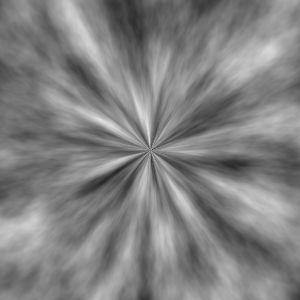Hehhey, noice. And notice that it doesn't even have the black area around the unit circle. This is because the Perlin noise generator is tiling: if gives back the same value at (0.5; 0.7) and (0.5;1.7).

Okay, a few more steps. First, the gradient. Now... this code is not the most optimal... or pretty... but as long as the rendering runs in seconds, and tweaking is easy, I don't actually care.

 ``` 1 2 3 4 5 6 7 8 9 10 11 12 13 14 15 16 17 18 19 20``` ``````def gradient(d, value): GRADIENTS = ( (0.35, 0.0), (0.4, 1.0), (0.55, value), (1.1, 0.0), ) for i in range(len(GRADIENTS) - 1): g0_d, g0_val = GRADIENTS[i] g1_d, g1_val = GRADIENTS[i + 1] if g1_d > d and g0_d <= d: r = (d - g0_d)/(g1_d - g0_d) r = r*r*(3 - 2*r) return g0_val + (g1_val - g0_val) * r return 0 def pixel_value(x, y): ... value = gradient(distance, value) return [value, value, value, 1] ``````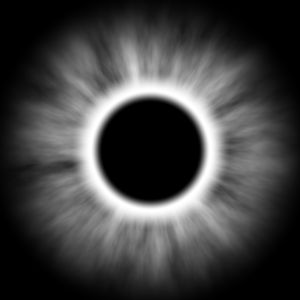Very spooky. We are at the final stretch. I added a non-linear twirl to it. With a simple twirl, you just add the distance to the angle and that's it, you get a spiral. With this 0.3th power, we can simulate the "virtual material" acquiring speed.

 ```1 2 3 4 5 6``` ``````def pixel_value(x, y): ... value = complex_noise( angle / (2*math.pi) + distance ** 0.3, distance, ... ``````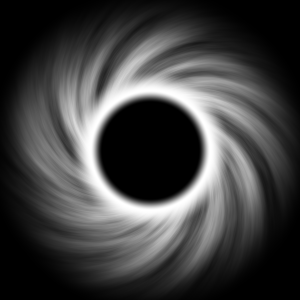And finally, the color mapping. We want to keep the white white (so value=1 should be RGB(1,1,1)), the middle somewhat purple, and the tails bluish. There are many ways to do it, I did it by playing around with powers:

 ```1 2 3 4 5 6``` ``````def color_map(value): return [value**2, value**3, value ** 1.5, 1] def pixel_value(x, y): ... return color_map(gradient(distance, value)) ``````And we are basically done. We pulled a void logo out of the void. :)

## Final code

The full code for the blogpost (freely usable, WTFPL licence) is found here:

 ``` 1 2 3 4 5 6 7 8 9 10 11 12 13 14 15 16 17 18 19 20 21 22 23 24 25 26 27 28 29 30 31 32 33 34 35 36 37 38 39 40 41 42 43 44 45 46 47 48 49 50 51 52 53 54 55 56 57 58 59 60``` ``````from perlin import complex_noise import png import math SIZE = 300 def polar_coords(x, y): x2 = x - 0.5 y2 = y - 0.5 angle = math.atan2(x2, y2) distance = math.sqrt(x2*x2 + y2*y2) return (angle, distance) def gradient(d, value): GRADIENTS = ( (0.16, 0.0), (0.2, 1.0), (0.27, value), (0.55, 0.0), ) for i in range(len(GRADIENTS) - 1): g0_d, g0_val = GRADIENTS[i] g1_d, g1_val = GRADIENTS[i + 1] if g1_d > d and g0_d <= d: r = (d - g0_d)/(g1_d - g0_d) r = r*r*(3 - 2*r) return g0_val + (g1_val - g0_val) * r return 0 def color_map(value): return [value**2, value**3, value ** 1.5, 1] def pixel_value(x, y): angle, distance = polar_coords(x, y) value = complex_noise( angle / (2*math.pi) + distance ** 0.3, distance, x_freq=20, y_freq=3, )*0.5 + 0.5 return color_map(gradient(distance, value)) def generate_image(): return [ b"".join( bytes( round(255 * component) for component in pixel_value(x/SIZE, y/SIZE) ) for x in range(SIZE) ) for y in range(SIZE) ] png.from_array(generate_image(), 'RGBA').save('out.png') ``````

## Outro

Animating it is left as an exercise to the reader. Hint: scrolling and tiling play very well with this code. Once you have the pngs (preferably numbered), and you did the alpha channel right (notice how it's 1 in the above code) the magic ffmpeg command to make a transparent webm is this:

 `1` ``````ffmpeg -framerate 30 -pattern_type glob -i '*.png' -c:v libvpx-vp9 -crf 12 -preset slower -pix_fmt yuva420p out.webm ``````

Oh on a related note, if you are rendering text with python, be careful with the example codes.

I forgot that I love texture generation. It's the quintessential demoscene skill. Go take a look at kkrieger or any of the 64k demoscenes sometimes, it's amazing what you can do procedurally.

Next article:
IMU prediction

If you need Augmented Reality problem solving, or want help implementing an AR or VR idea, drop us a mail at info@voidcomputing.hu